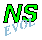## A dipole encounters a monopole

#### monopole weaker / encounter with offset

A Lamb dipole with radius 0.75 and velocity of +2 (i.e. moving to the right) is placed to the left of a positive Bessel monopole with radius 0.50 at the origin.

In the main run of this example the dipole is along the x-axis, making the encounter head-on, and the monpole has a circulation of +4, which means that the monopole is weaker than each dipole half.

In this example:

• The circulation of the monopole is +4, which makes the monopole weaker than each dipole half, since the circulation per dipole half is about 10.2.
• The dipole is located 0.5 above the x-axis: the encounter is with an offset.
The initial situation is shown in the following two graphs:Initial tracer (left) and vorticity (right) distribution.
The domain shown is x=[-3.0:+3.0], y=[-2.0:+2.0].
The extrema of voriticity are +/- 29.5 for the dipole
and +11.8 for the monopole.
The monopole has an angular momentum: it rotates about its central point, without moving. The dipole has a linear momentum: it moves in the direction of its axis, in this case to the right, towards the (weaker) monopole.

The monopole rotates counter-clockwise, like the top half of the dipole. The result of the rotation is that the dipole is pushed down, to negative y-values, while it moves towards the monopole, like in the main run without offset. But since the dipole now starts at a higher initial y-value, the dipole reaches the monopole with its negative half, rather than with its positive half. This can be seen clearest in the tracer plots: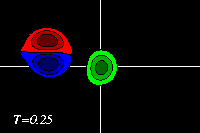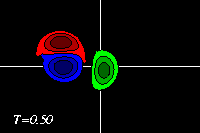The negative dipole half (blue) then forms with the positive monopole (green) a dipolar structure. Since the monopole is weaker than the dipole half, the newly formed dipolar structure will move along a curved path, with the stonger half on the inside. Meanwhile the original positive dipole half (red) is not a monopole, which does not move on its own (there is a small motion, due to the dipolar structure close by):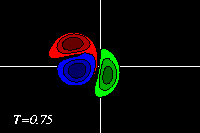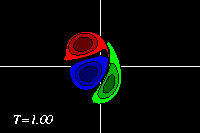The curved path of the dipolar structure brings the negative dipole half back close to its former positive and equally-strong partner. Their combination is so strong that the negative dipole half teams up again with its former partner to form a dipole. This dipole then moves away again from the weaker positive monopole. Due to the interaction, however, there is some shedding for vorticity and the monopole (green) has a small patch of negative vorticity (blue) from the dipole.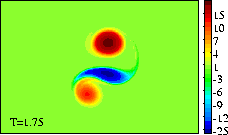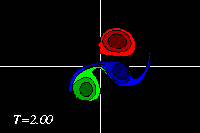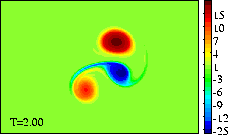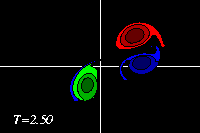In the main run without offset the monopole merges with the dipole into a new, a-symmetric dipole.
In this example there is an "exchange of partners", but this is only temporarily, because the monopole is weaker than the dipole. If this simulation is done with a monopole that is nearly as strong as the dipole, then the "exchange of partners" is permanent: the dipole moves away to negative y, leaving the original positive dipole half (red) behind as a monopole.

These MPEG movies (101 frames; 568kb) show the evolution until T=2.5 more clearly:
===> MPEG movie of the tracer distribution
===> MPEG movie of the vorticity distribution

At initialisation, a single tracer particle was placed at the extrema of vorticity of both vortices. The next graph shows their trajectories:Trajectories of the tracers, initially placed at
the extrema of vorticity of the monopole (solid
line) and the dipole (dashed line).
Symbols are placed at intervals of T=0.25.

See the main run of this example, with a weaker monopole and a head-on encounter.

The evolution of the vorticity distribution is computed with a Finite Difference Method which solves the two-dimensional vorticity (Navier-Stokes) equation. Time and distances are given in dimensionless units.

===> Some details on the computation presented on this page for those who are interested.